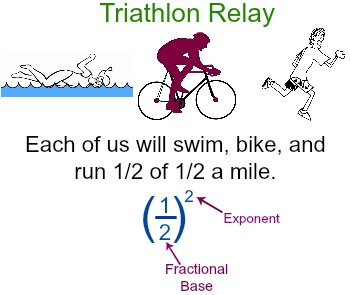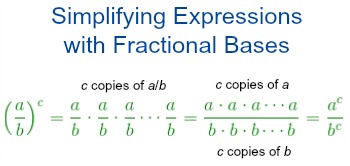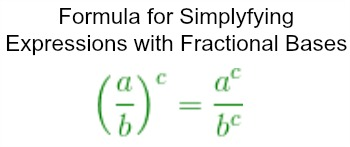# How to Simplify Expressions With Fractional Bases

Instructor: Laura Pennington

Laura has taught collegiate mathematics and holds a master's degree in pure mathematics.

Expressions with fractional bases can easily occur in the world around us. This lesson will look at how to simplify these types of expressions through vocabulary, definitions, and examples.

## Fractional Bases

Guess what!? Your school is putting on a fundraiser for an upcoming field trip, and it involves participating in a triathlon partner relay. You and your friend Mike decide to partner up. Let's raise some money!

The triathlon consists of a swimming section, a biking section, and a running section. Each section is half a mile long. Since this is a relay, you and Mike will each be doing half of the distance for each section. Let's figure out how far each of you will be swimming, biking, and running total.

Each section is 1 / 2 of a mile, and you are each doing 1 / 2 of that. So, we just have to multiply 1 / 2 by 1 / 2 to figure out our individual distances for each event. In other words, we need to square 1 / 2, or raise 1 / 2 to the power of 2.In mathematics, the expression (1 / 2)2 is called an exponential expression. In general, an exponential expression has the form:

• x y

We call x the base of the expression, and y the exponent. To evaluate this expression, we multiply x by itself y times.

Notice that in our example, (1 / 2)2, our base is a fraction. When the base of an exponential expression is a fraction, we call it a fractional base.

Now we know what all the parts of our expression are called, but how do we simplify it to figure out how far you and your partner will each be swimming, biking, and running? Let's figure it out.

## Simplifying Expressions with Fractional Bases

Let's consider a general exponential expression with a fractional base.

• (a / b)c

As it turns out, there is a nice formula for simplifying this expression. We could just jump straight into using that formula, but where's the fun in that? Let's see if we can find the formula ourselves and then use it.

As we said, we simplify an exponential expression, x y, by multiplying x by itself y times. Therefore, to simplify (a / b)c, we multiply a / b by itself c times. That's not so hard as long as we keep in mind that multiplying fractions simply involves multiplying the numerators together and the denominators together.We see that we end up with a fraction with a numerator of a multiplied by itself c times, or ac, and a denominator of b multiplied by itself c times, or bc. Basically, to raise a fractional base to an exponent, we raise both the numerator and denominator of the base to that exponent.Awesome! We have a formula we can use to simplify expressions with fractional bases!

For practice, let's look at the distances for the triathlon. We can use our formula to figure out how far you and your partner will swim, bike, and run.

 (1 / 2)2 Use the formula 12 / 22 Simplify (1 ⋅ 1) / (2 ⋅ 2) Multiply out 1 / 4 Simplified as much as possible

It looks like you will each be swimming 1 / 4 of a mile, biking 1 / 4 of a mile, and running 1 / 4 of a mile.

To unlock this lesson you must be a Study.com Member.

### Register to view this lesson

Are you a student or a teacher?

### Unlock Your Education

#### See for yourself why 30 million people use Study.com

##### Become a Study.com member and start learning now.
Back
What teachers are saying about Study.com

### Earning College Credit

Did you know… We have over 160 college courses that prepare you to earn credit by exam that is accepted by over 1,500 colleges and universities. You can test out of the first two years of college and save thousands off your degree. Anyone can earn credit-by-exam regardless of age or education level.